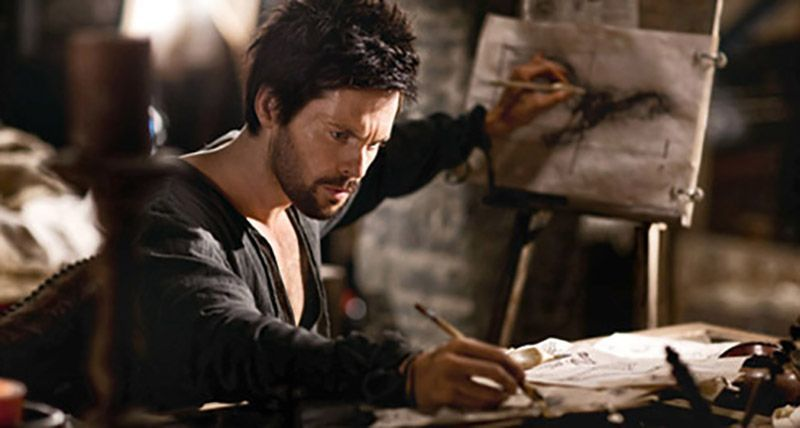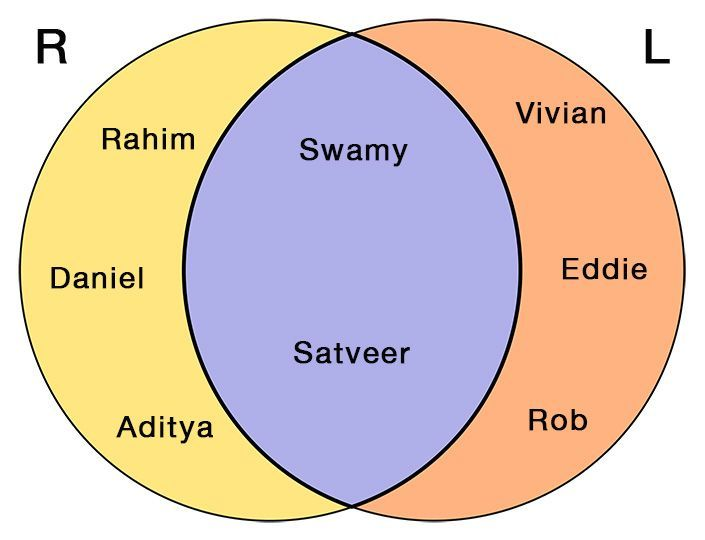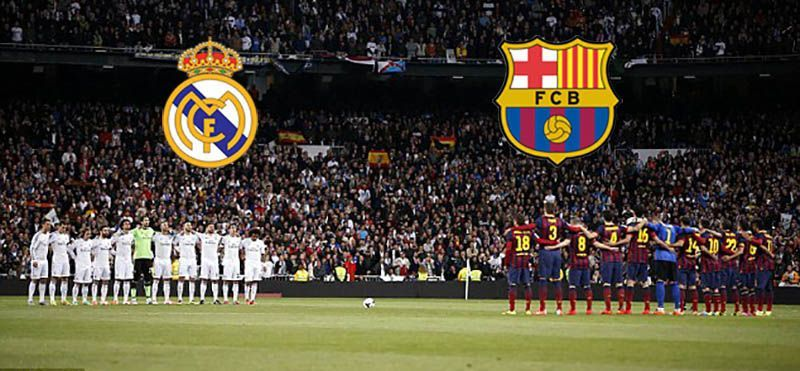# Writing with Both Hands!

The results of a survey done about the dominant hands of 8 people are shown below.

Set R, shown below, were dominant with Right Hand.

R = {Swamy, Rahim, Satveer, Daniel, Aditya}

Set L, shown below, were dominant with Left Hand.

L = {Vivian, Eddie, Swamy, Satveer, Rob}Represent them as a Venn Diagram.

We have 2 sets here and we have to represent them as Venn Diagrams.

So, the first thing that we need to do is, draw a Sets in a Venn Diagram.

# Venn Diagrams. Of 2 sets.

Intersecting sets are represented by overlapping circles.Draw a Rectangle, that encloses the set.

Draw a Circle/Ellipse for each of the set with elements in it.

# Access All Content

## More from Grade 4 Math# Pictograph

Representing your world in pictures! See how the type and number of a quantity can be mathematically represented pictorially.# Venn Diagram

The easiest way to imagine sets visually. See the power of Venn Diagrams.# Venn Diagram Example

Learn how to use Venn Diagrams to solve real world problems with these interesting examples.